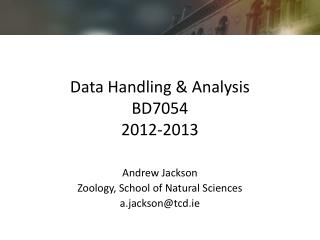# Data Handling & Analysis BD7054 2012-2013 - PowerPoint PPT PresentationDownload PresentationData Handling & Analysis BD7054 2012-2013

Download Presentation## Data Handling & Analysis BD7054 2012-2013

- - - - - - - - - - - - - - - - - - - - - - - - - - - E N D - - - - - - - - - - - - - - - - - - - - - - - - - - -
##### Presentation Transcript

1. Data Handling & AnalysisBD70542012-2013 Andrew Jackson Zoology, School of Natural Sciences a.jackson@tcd.ie

2. Introduction to Hypothesis Testing An Experiment Flipping coins

3. A simple experiment • Question: • Does adding weight to a coin make it unfair? • Blu-tac added to head side • Need to construct testable hypotheses • The null hypothesis

4. Weighted coin toss • Toss the coin 10 times • What is the hypothesis about how you think your system will behave? • More likely to get heads • Less likely to get heads • Either more or less likely to get heads • What are the corresponding null hypotheses? • That the coin is fair

5. Behaviour of a fair coin • The model is a fair 50:50 coin • How do we generate information about how a fair coin behaves? • Toss an un-weighted coin 10 times and repeat

6. Behaviour of weighted coin • Compare the weighted coin against the expected behaviour of a fair coin • Question • How likely is it that our observed coin is fair?

7. Behaviour of weighted coin

8. Alternative hypotheses • HA: coin is more likely to produce heads • One-tailed test in right tail • HA: coin is less likely to produce heads • One-tailed test in left tail • HA: coin is unfair (in either direction) • Two-tailed test including both left and right tails

9. P-values • A p-value is the probability of your observed data or more extreme being generated according to the null hypothesis • The less likely your data are, the less likely you would accept the null hypothesis as being true • We generally use a cut-off of p<0.05 to accept the alternative hypothesis • One or two tailed tests refer to where you predict your alternative hypotheses to lie before you do your experiment

10. Summary • Science is about constructing experiments or designing observations to test your ideas about how the world works • Hypotheses must be falsifiable • Generally we construct null hypotheses against which our alternative hypotheses can be tested • p-values tell us how likely it is our data came from the null hypothesis and therefore allow us to accept or reject it (H0)